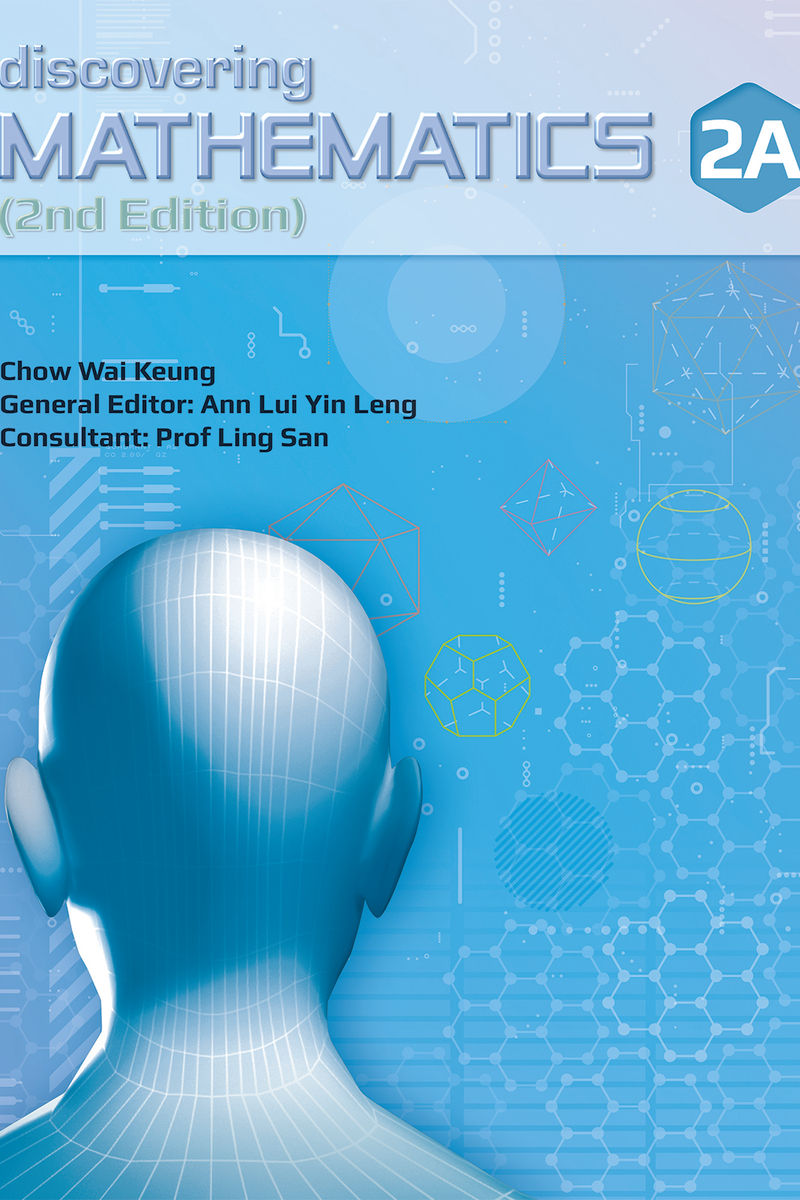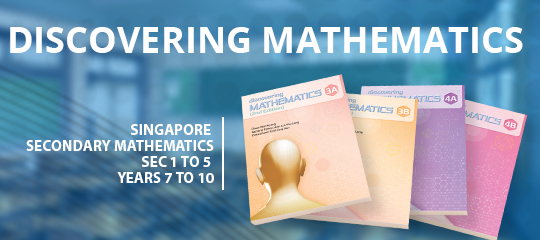Our prices are denominated in USD.

We ship internationally!# Discovering Mathematics Textbook 2A (2nd Edition)

\$16.20

Discovering Mathematics (2nd Edition) is a series designed for the students in secondary schools and approved by the Ministry of Education, Singapore, for the latest secondary Mathematics syllabus. The series prepares students for the Singapore Cambridge O-Level examinations, and guides students to understand and internalise mathematical concepts and apply them in real-life.

The emphasis of this series is on empowering students to learn mathematics independently and effectively. Depending on the topics covered, different approaches are used to present different concepts to facilitate students' understanding.

This series includes clear and illustrative examples, class activities and diagrams to help students understand the concepts and apply them.

Key features:

1. Chapter Opener
Introduces a chapter by establishing a connection between topic and real world situations. Learning objectives for the chapter are also provided.
2. Class Activities
Allows students to learn mathematical concepts through exploration and discovery
3. Examples and Try it!
Worked examples have been included to facilitate students' understanding of concepts and show how ideas and reasoning can be expressed clearly in mathematical language. Analyses and hints are given to illustrate problem solving skills. After each worked example, a Try it! is provided for students to practise a similar question and find out if they have understood the concept(s) presented earlier.
4. Exercises
Each section of a chapter includes an exercise for the students. The questions in each exercise have been sequenced as Level 1, 2 and 3. These questions encourage students to think analytically, reason and communicate effectively in mathematical language.
Level 1: Simple questions involving direct application of the concepts
Level 2: More challenging questions on direct applications
Level 3: Questions that involve real-life applications, thinking skills or presentation of open-ended answers
5. Review Exercises
A Review Exercise is inserted after several chapters. This allows students to check their mastery of the concepts that have been learnt.
6. Problem-solving Processes and Heuristics
7. Problems in Real-world Contexts
8. In a Nutshell
Summarises the important concepts in each chapter to help students recap and revise them.
At the end of each chapter, students will be encouraged to write about their learning experience.
10. Remark
Some important information that is of interest to students has been included.
11. Recall
Concepts or definitions covered earlier have been included to help students recall and relate them to topics being studies.
12. Discuss
Questions concerning the concepts being taught have been included to encourage meaningful interaction between the teacher and the students.
13. Spot Check
Some short questions are posed to help students clarify the concept(s) being taught.
14. Maths Matter
Interesting facts related to mathematics, mathematicians and history of mathematics, are presented to make the learning process more enriching.

For Secondary 2 / Grade 8 / Year 8 / 14 years old

Singapore Curriculum

1. Proportion
Map Scale, Direction and Inverse Proportion
2. Expansion and Factorisation of Algebraic Expressions
Expansion and Factorisation of Quadratic Expressions
3. Simple Algebraic Fractions
Simplification, Addition, Subtraction, Multiplication and Division of Algebraic Fractions, Changing Subject of a Formula
Graphs, Solving and Application of Quadratic Equations
5. Linear Equations in Two Variables
Solving Simultaneous Equations by Graphical, Substitution and Elimination Methods
6. Congruency and Similarity
Congruency, Similarity, Scale Factor and Scale Drawing

For topics in this book, click here.

Complete series contain:

1. Textbook
2. Workbook
3. Workbook Solution
4. Question Bank
5. Teachers' Guide

See all here.